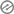# Explain the concept of significance level.

Explain the concept of significance level. Scientific conclusions are statements with high probability of being correct and not statements of absolute truth. Before we carry out the experiment, we must determine how high the probability will be in order to accept or reject a null hypothesis. We need to carry out a test of significance before we can decide whether to reject or accept a null hypothesis. Based on sample data, the test of significance determines whether the difference is significantly greater than a chance difference resulting from sampling error.

Absolutely, the significance level is a critical concept in hypothesis testing. It represents the threshold below which you’re willing to accept that a result occurred by chance alone. It’s often denoted by the symbol “α” and is usually set prior to conducting an experiment.

When conducting a hypothesis test, you compare the p-value (the probability of obtaining a result as extreme as the one observed, assuming the null hypothesis is true) with the significance level. If the p-value is less than or equal to the significance level, you reject the null hypothesis in favor of the alternative hypothesis. This decision is based on the idea that the result is unlikely to have occurred by random chance alone.

However, as you rightly mentioned, scientific conclusions are not absolute truths. The significance level you choose (commonly 0.05 or 0.01) helps control the rate of making Type I errors (rejecting a true null hypothesis), but it doesn’t guarantee that a significant result is practically important or replicable. It’s important to interpret results in the context of the field, experiment design, and potential sources of bias.

[mediator_tech]Using a scatter diagram differentiate between negative, positive and zero correlation.• Significance Level: The threshold for deciding how much evidence is needed before accepting or rejecting a hypothesis.
• Scientific Conclusions: Statements that are highly probable but not absolute truths; they’re based on evidence and analysis.
• Hypothesis Testing: Before an experiment, determine the probability threshold (significance level) for accepting or rejecting a null hypothesis.
•• Test of Significance: An analysis carried out after data collection to assess if the observed difference is significantly greater than chance due to sampling error.
• P-value Comparison: Compare the p-value (probability of extreme results under null hypothesis) with the significance level.
• Reject or Accept: If p-value ≤ significance level, reject null hypothesis; if p-value > significance level, accept null hypothesis.
•• Control Type I Errors: Significance level helps control the risk of wrongly rejecting a true null hypothesis.
• Interpretation Needed: Significant results aren’t absolute; consider experimental design, field context, and biasesEvaluation

1. The significance level is the threshold for deciding how much evidence is needed before __________ a hypothesis.
a) accepting
b) rejecting
c) formulating

2. Scientific conclusions are statements that carry a high probability of being correct but are not __________ truths.
a) absolute
b) relative
c) conditional3. A test of significance is carried out after data collection to determine whether the observed difference is significantly greater than __________.
a) theoretical expectations
b) chance due to sampling error
c) previous studies

4. The probability of obtaining a result as extreme as the observed one, assuming the null hypothesis is true, is known as the __________.
a) margin of error
b) p-value
c) confidence interval

5. The comparison between the p-value and the significance level helps in deciding whether to __________ the null hypothesis.
a) accept
b) reject
c) modify6. The significance level helps control the rate of making __________ errors, where a true null hypothesis is rejected.
a) Type I
b) Type II
c) Type III

7. A lower significance level (α) indicates that you are requiring __________ evidence to reject the null hypothesis.
a) stronger
b) weaker
c) identical

8. Hypothesis testing involves comparing observed data with __________.
a) personal beliefs
b) anecdotal evidence
c) null hypothesis predictions9. The practical importance and __________ of a significant result should be considered in addition to statistical significance.
a) complexity
b) context
c) confidentiality

10. A p-value less than or equal to the significance level leads to the decision to __________ the null hypothesis.
a) accept
b) reject
c) modify

11. Setting a significance level prior to conducting an experiment helps prevent __________ decision-making based on the data.
a) hasty
b) consistent
c) delayed12. The significance level is often denoted by the symbol __________.
a) δ
b) β
c) α

13. Significance level controls the balance between making __________ errors and missing true effects.
a) Type I
b) Type II
c) both Type I and Type II

14. Scientific conclusions based on significance testing are not absolute truths because they depend on the __________.
a) funding source
b) research institute
c) available evidence15. The choice of significance level is a trade-off between __________.
a) credibility and speed
b) complexity and simplicity
c) rejection and acceptance